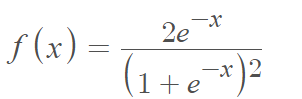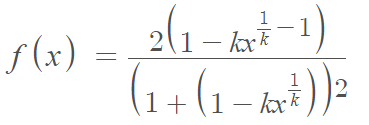# Half-Logistic Distribution

Share on

The half-logistic distribution is a one parameter, continuous probability distribution created by folding the standard logistic distribution (this leads to its alternate name, the folded logistic distribution) ; It is equivalent to a logistic distribution with only positive values and a zero mean.

This widely used classical distribution is popular for modeling failure times of components; It has decreasing density and increasing failure rate  with a reliability function of R(t) = 2 / (1 + ebt).

## PDF of the Half-Logistic Distribution

The probability density function for the half-logistic distribution isfor x ≥ 0.

## Extensions of the Half-Logistic Distribution

Although widely used in a variety of fields, the half logistic distribution does have one major disadvantage— it cannot model data with non-monotone failure rates and unimodal density . To address this, many extensions have been proposed including the power half logistic , Olapade-half logistic, and the generalized half logistic, each with their own PDFs. For example, the generalized version has PDF## References

Balakrishnan, N. (1991). Handbook of the Logistic Distribution. Taylor & Francis.
 Muhammad, M. & Liu, L. A New Extension of the Generalized Half Logistic Distribution with Applications to Real Data. Entropy (Basel). 2019 Apr; 21(4): 339.
Published online 2019 Mar 28. doi: 10.3390/e21040339